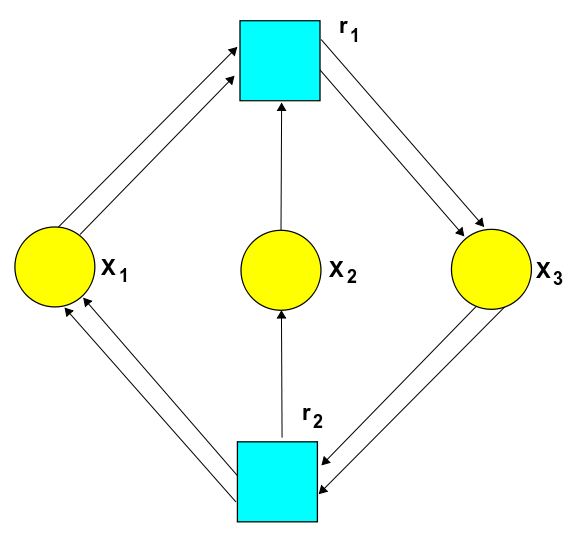# The Azimuth Project Experiments in bimolecular reactions (Rev #2)

### A bimolecular reaction

For the general terms and definitions used in this project see

Here we will consider an example of a bimolecular reaction. This is example 6.3 in the paper

From Equation (12) page 13 we have the following chemical reaction network.

$k_1 : 2 X_1 + X_2 \rightarrow 2 X_3$
$k_2: 2 X_3 \rightarrow 2 X_1 + X_2$

### The stochastic Petri net### The rate equation

$\frac{d}{d t} X_1 = -2 k_1 X_1^2 X_2 + 2 k_2 X_3^2$
$\frac{d}{d t} X_2 = - k_1 X_1^2 X_2 + k_2 X_3^2$
$\frac{d}{d t} X_3 = 2 k_1 X_1^2 X_2 + -2 k_2 X_3^2$

### The master equation

$H = k_1(a_3^\dagger a_3^\dagger a_1 a_1 a_2 - a_1^\dagger a_1^\dagger a_1 a_1 a_2^\dagger a_2) + k_2 (a_1^\dagger a_1^\dagger a_2^\dagger -a_3^\dagger a_3^\dagger a_3 a_3)$

From this we arrive at the following

$z_3^2; 0 = k_1 c_1^2 c_2 - k_2 c_3^2$
$z_1^2 z_2; 0 = k_2 c_3^2 - k_1 c_1^2 c_2$

category: experiments